# Pandas教程（非常详细）

### 文章目录

• 教程特点
• 阅读条件
• Pandas是什么
• Pandas主要特点
• Pandas主要优势
• Pandas内置数据结构
• Pandas库下载和安装
• Windows系统安装
• Linux系统安装
• 1) Ubuntu用户
• 2) Fedora用户
• MacOSX系统安装
• Pandas Series入门教程
• 创建Series对象
• 1) 创建一个空Series对象
• 2) ndarray创建Series对象
• 3) dict创建Series对象
• 4) 标量创建Series对象
• 访问Series数据
• 1) 位置索引访问
• 2) 索引标签访问
• Series常用属性
• 1) axes
• 2) dtype
• 3) empty
• 4) ndim
• 5) size
• 6) values
• 7) index
• Series常用方法
• 2) isnull()&nonull()检测缺失值
• Pandas DataFrame入门教程（图解版）
• 认识DataFrame结构
• 创建DataFrame对象
• 1) 创建空的DataFrame对象
• 2) 列表创建DataFame对象
• 3) 字典嵌套列表创建
• 4) 列表嵌套字典创建DataFrame对象
• 5) Series创建DataFrame对象
• 列索引操作DataFrame
• 1) 列索引选取数据列
• 2) 列索引添加数据列
• 3) 列索引删除数据列
• 行索引操作DataFrame
• 1) 标签索引选取
• 2) 整数索引选取
• 3) 切片操作多行选取
• 4) 添加数据行
• 5) 删除数据行
• 常用属性和方法汇总
• 1) T（Transpose）转置
• 2) axes
• 3) dtypes
• 4) empty
• 5) ndim
• 6) shape
• 7) size
• 8) values
• 10) shift()移动行或列
• Pandas Panel三维数据结构
• pandas.Panel()
• 创建Panel 对象
• 1) 创建一个空Panel
• 2) ndarray三维数组创建
• 3) DataFrame创建
• Panel中选取数据
• 1) 使用 items选取数据
• Python Pandas描述性统计
• sum()求和
• mean()求均值
• std()求标准差
• 数据汇总描述
• Python Pandas绘图教程（详解版）
• 柱状图
• 直方图
• 箱型图
• 区域图
• 散点图
• 饼状图
• Pandas csv读写文件
• 1) 自定义索引
• 2) 查看每一列的dtype
• 3) 更改文件标头名
• 4) 跳过指定的行数
• to_csv()
• Pandas Excel读写操作详解
• to_excel()
• Pandas和NumPy的比较
• 创建数组
• 布尔索引
• 重塑数组形状
• Pdans与NumPy区别
• 转换ndarray数组
• Pandas 库是一个免费、开源的第三方 Python 库，是 Python 数据分析必不可少的工具之一，它为 Python 数据分析提供了高性能，且易于使用的数据结构，即 Series 和 DataFrame。Pandas 自诞生后被应用于众多的领域，比如金融、统计学、社会科学、建筑工程等。

Pandas 库基于 Python NumPy 库开发而来，因此，它可以与 Python 的科学计算库配合使用。Pandas 提供了两种数据结构，分别是 Series（一维数组结构）与 DataFrame（二维数组结构），这两种数据结构极大地增强的了 Pandas 的数据分析能力。在本套教程中，我们将学习 Python Pandas 的各种方法、特性以及如何在实践中运用它们。

## 教程特点

本套教程是为 Pandas 初学者打造的，学习完本套教程，您将在一定程度上掌握 Pandas 的基础知识，以及各种功能。如果您是从事数据分析的工作人员，那么这套教程会对您有所帮助。

本套教程对 Python Pandas 库进行详细地讲解，包括文件读写、统计学函数、缺失值处理、以及数据可视化等重点知识。为了降低初学者的学习门槛，我们的教程尽量采用通俗易懂、深入浅出的语言风格，相信通过对本套教程的学习，您一定会收获颇丰。

## 阅读条件

在开始学习本套教程前，首先您应该对于数据分析、数据可视化的概念有一定程度的了解，并且您已经熟练掌握 Python 语言的基础知识。其次，由于 Pandas 库是在 NumPy 库的基础上构建而来，所以建议您提前学习《Python NumPy教程》。

# Pandas是什么

Pandas 是一个开源的第三方 Python 库，从 Numpy 和 Matplotlib 的基础上构建而来，享有数据分析“三剑客之一”的盛名（NumPy、Matplotlib、Pandas）。Pandas 已经成为 Python 数据分析的必备高级工具，它的目标是成为强大、灵活、可以支持任何编程语言的数据分析工具。图1：Pandas Logo

Pandas 这个名字来源于面板数据（Panel Data）与数据分析（data analysis）这两个名词的组合。在经济学中，Panel Data 是一个关于多维数据集的术语。Pandas 最初被应用于金融量化交易领域，现在它的应用领域更加广泛，涵盖了农业、工业、交通等许多行业。

Pandas 最初由 Wes McKinney（韦斯·麦金尼）于 2008 年开发，并于 2009 年实现开源。目前，Pandas 由 PyData 团队进行日常的开发和维护工作。在 2020 年 12 月，PyData 团队公布了最新的 Pandas 1.20 版本 。

在 Pandas 没有出现之前，Python 在数据分析任务中主要承担着数据采集和数据预处理的工作，但是这对数据分析的支持十分有限，并不能突出 Python 简单、易上手的特点。Pandas 的出现使得 Python 做数据分析的能力得到了大幅度提升，它主要实现了数据分析的五个重要环节：

• 加载数据
• 整理数据
• 操作数据
• 构建数据模型
• 分析数据
• ## Pandas主要特点

Pandas 主要包括以下几个特点：

• 它提供了一个简单、高效、带有默认标签（也可以自定义标签）的 DataFrame 对象。
• 能够快速得从不同格式的文件中加载数据（比如 Excel、CSV 、SQL文件），然后将其转换为可处理的对象；
• 能够按数据的行、列标签进行分组，并对分组后的对象执行聚合和转换操作；
• 能够很方便地实现数据归一化操作和缺失值处理；
• 能够很方便地对 DataFrame 的数据列进行增加、修改或者删除的操作；
• 能够处理不同格式的数据集，比如矩阵数据、异构数据表、时间序列等；
• 提供了多种处理数据集的方式，比如构建子集、切片、过滤、分组以及重新排序等。
• 上述知识点将在后续学习中为大家一一讲解。

## Pandas主要优势

与其它语言的数据分析包相比，Pandas 具有以下优势：

• Pandas 的 DataFrame 和 Series 构建了适用于数据分析的存储结构；
• Pandas 简洁的 API 能够让你专注于代码的核心层面；
• Pandas 实现了与其他库的集成，比如 Scipy、scikit-learn 和 Matplotlib；
• Pandas 官方网站（点击访问）提供了完善资料支持，及其良好的社区环境。
• ## Pandas内置数据结构

我们知道，构建和处理二维、多维数组是一项繁琐的任务。Pandas 为解决这一问题， 在 ndarray 数组（NumPy 中的数组）的基础上构建出了两种不同的数据结构，分别是 Series（一维数据结构）DataFrame（二维数据结构）：

• Series 是带标签的一维数组，这里的标签可以理解为索引，但这个索引并不局限于整数，它也可以是字符类型，比如 a、b、c 等；
• DataFrame 是一种表格型数据结构，它既有行标签，又有列标签。
• 下面对上述数据结构做简单地的说明：

数据结构 维度 说明
Series 1 该结构能够存储各种数据类型，比如字符数、整数、浮点数、Python 对象等，Series 用 name 和 index 属性来描述 数据值。Series 是一维数据结构，因此其维数不可以改变。
DataFrame 2 DataFrame 是一种二维表格型数据的结构，既有行索引，也有列索引。行索引是 index，列索引是 columns。 在创建该结构时，可以指定相应的索引值。

由于上述数据结构的存在，使得处理多维数组数任务变的简单。在《Pandas Series入门教程》和《Pandas DataFrame入门教程（图解）》两节中，我们会对上述数据结构做详细讲解。

注意，在 Pandas 0.25 版本后，Pamdas 废弃了 Panel 数据结构，如果感兴趣可阅读《Pandas Panel三维数据结构》一节。

# Pandas库下载和安装

Python 官方标准发行版并没有自带 Pandas 库，因此需要另行安装。除了标准发行版外，还有一些第三方机构发布的 Python 免费发行版， 它们在官方版本的基础上开发而来，并有针对性的提前安装了一些 Python 模块，从而满足某些特定领域的需求，比如专门适应于科学计算领域的 Anaconda，它就提前安装了多款适用于科学计算的软件包。

对于第三方发行版而言，它们已经自带 Pandas 库，所以无须另行安装。下面介绍了常用的免费发行版：

1. Anaconda（官网下载：https://www.anaconda.com/）是一个开源的 Python 发行版，包含了 180 多个科学包及其依赖项。除了支持 Windows 系统外，也支持 Linux 和 Mac 系统。

2. Python(x,y)（下载地址：https://python-xy.github.io/）是一款基于 Python、Qt （图形用户界面）和 Spyder （交互式开发环境）开发的软件，主要用于数值计算、数据分析和数据可视化等工程项目，目前只支持 Python 2 版本。

3. WinPython（下载地址：https://sourceforge.net/projects/winpython/files/）一个免费的 Python 发行版，包含了常用的科学计算包与 Spyder IDE，但仅支持 Windows 系统。

下面介绍在不同操作系统环境下，标准发行版安装 Pandas 的方法。

## Windows系统安装

使用 pip 包管理器安装 Pandas，是最简单的一种安装方式。在 CMD 命令提示符界面行执行以下命令：

pip install pandas

## Linux系统安装

对于不同的版本的 Linux 系统，您可以采用它们各自的包管理器来安装 Pandas。

#### 1) Ubuntu用户

Pandas 通常需要与其他软件包一起使用，因此可采用以下命令，一次性安装所有包：

``````sudo apt-get install numpy scipy matplotlib pandas
``````

#### 2) Fedora用户

对于 Fedora 用户而言，可采用以下命令安装：

``````sudo yum install numpy scipy matplotlib pandas
``````

## MacOSX系统安装

对于 Mac 用户而言，同样可以直接使用 pip 包管理器来安装，命令如下：

``````pip install pandas
``````

# Pandas Series入门教程

Series 结构，也称 Series 序列，是 Pandas 常用的数据结构之一，它是一种类似于一维数组的结构，由一组数据值（value）和一组标签组成，其中标签与数据值之间是一一对应的关系。

Series 可以保存任何数据类型，比如整数、字符串、浮点数、Python 对象等，它的标签默认为整数，从 0 开始依次递增。Series 的结构图，如下所示：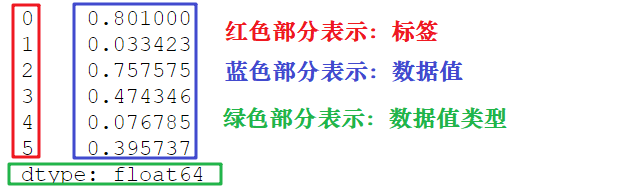通过标签我们可以更加直观地查看数据所在的索引位置。

## 创建Series对象

Pandas 使用 Series() 函数来创建 Series 对象，通过这个对象可以调用相应的方法和属性，从而达到处理数据的目的：

``````import pandas as pd
s=pd.Series( data, index, dtype, copy)
``````

参数说明如下所示：

参数名称 描述
data 输入的数据，可以是列表、常量、ndarray 数组等。
index 索引值必须是惟一的，如果没有传递索引，则默认为 np.arrange(n)。
dtype dtype表示数据类型，如果没有提供，则会自动判断得出。
copy 表示对 data 进行拷贝，默认为 False。

我们也可以使用数组、字典、标量值或者 Python 对象来创建 Series 对象。下面展示了创建 Series 对象的不同方法：

#### 1) 创建一个空Series对象

使用以下方法可以创建一个空的 Series 对象，如下所示：

``````import pandas as pd
#输出数据为空
s = pd.Series()
print(s)
``````

输出结果如下：

Series([], dtype: float64)

#### 2) ndarray创建Series对象

ndarray 是 NumPy 中的数组类型，当 data 是 ndarry 时，传递的索引必须具有与数组相同的长度。假如没有给 index 参数传参，在默认情况下，索引值将使用是 range(n) 生成，其中 n 代表数组长度，如下所示：

[0,1,2,3…. range(len(array))-1]

使用默认索引，创建 Series 序列对象：

``````import pandas as pd
import numpy as np
data = np.array(['a','b','c','d'])
s = pd.Series(data)
print (s)
``````

输出结果如下：

``````0   a
1   b
2   c
3   d
dtype: object
``````

上述示例中没有传递任何索引，所以索引默认从 0 开始分配 ，其索引范围为 0 到`len(data)-1`，即 0 到 3。这种设置方式被称为“隐式索引”。

除了上述方法外，你也可以使用“显式索引”的方法定义索引标签，示例如下：

``````import pandas as pd
import numpy as np
data = np.array(['a','b','c','d'])
#自定义索引标签（即显示索引）
s = pd.Series(data,index=[100,101,102,103])
print(s)
``````

输出结果：

``````100  a
101  b
102  c
103  d
dtype: object
``````

#### 3) dict创建Series对象

您可以把 dict 作为输入数据。如果没有传入索引时会按照字典的键来构造索引；反之，当传递了索引时需要将索引标签与字典中的值一一对应。

下面两组示例分别对上述两种情况做了演示。

示例1，没有传递索引时：

``````import pandas as pd
import numpy as np
data = {'a' : 0., 'b' : 1., 'c' : 2.}
s = pd.Series(data)
print(s)
``````

输出结果：

``````a 0.0
b 1.0
c 2.0
dtype: float64
``````

示例 2，为`index`参数传递索引时：

``````import pandas as pd
import numpy as np
data = {'a' : 0., 'b' : 1., 'c' : 2.}
s = pd.Series(data,index=['b','c','d','a'])
print(s)
``````

输出结果：

``````b 1.0
c 2.0
d NaN
a 0.0
dtype: float64
``````

当传递的索引值无法找到与其对应的值时，使用 NaN（非数字）填充。

#### 4) 标量创建Series对象

如果 data 是标量值，则必须提供索引，示例如下：

``````import pandas as pd
import numpy as np
s = pd.Series(5, index=[0, 1, 2, 3])
print(s)
``````

输出如下：

``````0  5
1  5
2  5
3  5
dtype: int64
``````

标量值按照 index 的数量进行重复，并与其一一对应。

## 访问Series数据

上述讲解了创建 Series 对象的多种方式，那么我们应该如何访问 Series 序列中元素呢？分为两种方式，一种是位置索引访问；另一种是索引标签访问。

#### 1) 位置索引访问

这种访问方式与 ndarray 和 list 相同，使用元素自身的下标进行访问。我们知道数组的索引计数从 0 开始，这表示第一个元素存储在第 0 个索引位置上，以此类推，就可以获得 Series 序列中的每个元素。下面看一组简单的示例：

``````import pandas as pd
s = pd.Series([1,2,3,4,5],index = ['a','b','c','d','e'])
print(s)  #位置下标
print(s['a']) #标签下标
``````

输出结果：

1
1

通过切片的方式访问 Series 序列中的数据，示例如下：

``````import pandas as pd
s = pd.Series([1,2,3,4,5],index = ['a','b','c','d','e'])
print(s[:3])
``````

输出结果：

``````a  1
b  2
c  3
dtype: int64
``````

如果想要获取最后三个元素，也可以使用下面的方式：

``````import pandas as pd
s = pd.Series([1,2,3,4,5],index = ['a','b','c','d','e'])
print(s[-3:])
``````

输出结果：

``````c  3
d  4
e  5
dtype: int64
``````

#### 2) 索引标签访问

Series 类似于固定大小的 dict，把 index 中的索引标签当做 key，而把 Series 序列中的元素值当做 value，然后通过 index 索引标签来访问或者修改元素值。

示例1，使用索标签访问单个元素值：

``````import pandas as pd
s = pd.Series([6,7,8,9,10],index = ['a','b','c','d','e'])
print(s['a']）
``````

输出结果：

6

示例 2，使用索引标签访问多个元素值

``````import pandas as pd
s = pd.Series([6,7,8,9,10],index = ['a','b','c','d','e'])
print(s[['a','c','d']])
``````

输出结果：

``````a    6
c    8
d    9
dtype: int64
``````

示例3，如果使用了 index 中不包含的标签，则会触发异常：

``````import pandas as pd
s = pd.Series([6,7,8,9,10],index = ['a','b','c','d','e'])
#不包含f值
print(s['f'])
``````

输出结果：

``````......
KeyError: 'f'
``````

## Series常用属性

下面我们介绍 Series 的常用属性和方法。在下表列出了 Series 对象的常用属性。

名称 属性
axes 以列表的形式返回所有行索引标签。
dtype 返回对象的数据类型。
empty 返回一个空的 Series 对象。
ndim 返回输入数据的维数。
size 返回输入数据的元素数量。
values 以 ndarray 的形式返回 Series 对象。
index 返回一个RangeIndex对象，用来描述索引的取值范围。

现在创建一个 Series 对象，并演示如何使用上述表格中的属性。如下所示：

``````import pandas as pd
import numpy as np
s = pd.Series(np.random.randn(5))
print(s)
``````

输出结果：

``````0    0.898097
1    0.730210
2    2.307401
3   -1.723065
4    0.346728
dtype: float64
``````

上述示例的行索引标签是 [0,1,2,3,4]。

#### 1) axes

``````import pandas as pd
import numpy as np
s = pd.Series(np.random.randn(5))
print ("The axes are:")
print(s.axes)
``````

输出结果

``````The axes are:
[RangeIndex(start=0, stop=5, step=1)]
``````

#### 2) dtype

``````import pandas as pd
import numpy as np
s = pd.Series(np.random.randn(5))
print ("The dtype is:")
print(s.dtype)
``````

输出结果：

``````The dtype is:
float64
``````

#### 3) empty

返回一个布尔值，用于判断数据对象是否为空。示例如下：

``````import pandas as pd
import numpy as np
s = pd.Series(np.random.randn(5))
print("是否为空对象?")
print (s.empty)
``````

输出结果：

``````是否为空对象?
False
``````

#### 4) ndim

查看序列的维数。根据定义，Series 是一维数据结构，因此它始终返回 1。

``````import pandas as pd
import numpy as np
s = pd.Series(np.random.randn(5))
print (s)
print (s.ndim)
``````

输出结果：

``````0    0.311485
1    1.748860
2   -0.022721
3   -0.129223
4   -0.489824
dtype: float64
1
``````

#### 5) size

返回 Series 对象的大小(长度)。

``````import pandas as pd
import numpy as np
s = pd.Series(np.random.randn(3))
print (s)
#series的长度大小
print(s.size)
``````

输出结果：

``````0   -1.866261
1   -0.636726
2    0.586037
dtype: float64
3
``````

#### 6) values

以数组的形式返回 Series 对象中的数据。

``````import pandas as pd
import numpy as np
s = pd.Series(np.random.randn(6))
print(s)
print("输出series中数据")
print(s.values)
``````

输出结果：

``````0   -0.502100
1    0.696194
2   -0.982063
3    0.416430
4   -1.384514
5    0.444303
dtype: float64
输出series中数据
[-0.50210028  0.69619407 -0.98206327  0.41642976 -1.38451433  0.44430257]
``````

#### 7) index

该属性用来查看 Series 中索引的取值范围。示例如下：

``````#显示索引
import pandas as pd
s=pd.Series([1,2,5,8],index=['a','b','c','d'])
print(s.index)
#隐式索引
s1=pd.Series([1,2,5,8])
print(s1.index)
``````

输出结果：

``````隐式索引：
Index(['a', 'b', 'c', 'd'], dtype='object')
显示索引：
RangeIndex(start=0, stop=4, step=1)
``````

## Series常用方法

如果想要查看 Series 的某一部分数据，可以使用 head() 或者 tail() 方法。其中 head() 返回前 n 行数据，默认显示前 5 行数据。示例如下：

``````import pandas as pd
import numpy as np
s = pd.Series(np.random.randn(5))
print ("The original series is:")
print (s)
#返回前三行数据
``````

输出结果：

``````原系列输出结果:
0    1.249679
1    0.636487
2   -0.987621
3    0.999613
4    1.607751
dtype: float64
0    1.249679
1    0.636487
2   -0.987621
dtype: float64
``````

tail() 返回的是后 n 行数据，默认为后 5 行。示例如下：

``````import pandas as pd
import numpy as np
s = pd.Series(np.random.randn(4))
#原series
print(s)
#输出后两行数据
print (s.tail(2))
``````

输出结果：

``````原Series输出：
0    0.053340
1    2.165836
2   -0.719175
3   -0.035178
输出后两行数据：
dtype: float64
2   -0.719175
3   -0.035178
dtype: float64
``````

#### 2) isnull()&nonull()检测缺失值

isnull() 和 nonull() 用于检测 Series 中的缺失值。所谓缺失值，顾名思义就是值不存在、丢失、缺少。

• isnull()：如果为值不存在或者缺失，则返回 True。
• notnull()：如果值不存在或者缺失，则返回 False。
• 其实不难理解，在实际的数据分析任物中，数据的收集往往要经历一个繁琐的过程。在这个过程中难免会因为一些不可抗力，或者人为因素导致数据丢失的现象。这时，我们可以使用相应的方法对缺失值进行处理，比如均值插值、数据补齐等方法。上述两个方法就是帮助我们检测是否存在缺失值。示例如下：

``````import pandas as pd
#None代表缺失数据
s=pd.Series([1,2,5,None])
print(pd.isnull(s))  #是空值返回True
print(pd.notnull(s)) #空值返回False
``````

输出结果：

``````0    False
1    False
2    False
3     True
dtype: bool

notnull():
0     True
1     True
2     True
3    False
dtype: bool
``````

# Pandas DataFrame入门教程（图解版）

DataFrame 是 Pandas 的重要数据结构之一，也是在使用 Pandas 进行数据分析过程中最常用的结构之一，可以这么说，掌握了 DataFrame 的用法，你就拥有了学习数据分析的基本能力。

## 认识DataFrame结构

DataFrame 一个表格型的数据结构，既有行标签（index），又有列标签（columns），它也被称异构数据表，所谓异构，指的是表格中每列的数据类型可以不同，比如可以是字符串、整型或者浮点型等。其结构图示意图，如下所示：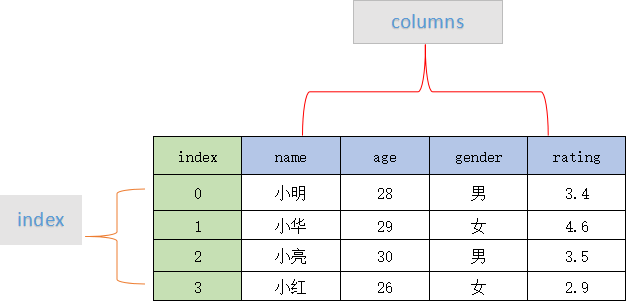表格中展示了某个销售团队个人信息和绩效评级（rating）的相关数据。数据以行和列形式来表示，其中每一列表示一个属性，而每一行表示一个条目的信息。

下表展示了上述表格中每一列标签所描述数据的数据类型，如下所示：

Column Type
name String
age integer
gender String
rating Float

DataFrame 的每一行数据都可以看成一个 Series 结构，只不过，DataFrame 为这些行中每个数据值增加了一个列标签。因此 DataFrame 其实是从 Series 的基础上演变而来。在数据分析任务中 DataFrame 的应用非常广泛，因为它描述数据的更为清晰、直观。

通过示例对 DataFrame 结构做进一步讲解。 下面展示了一张学生成绩表，如下所示：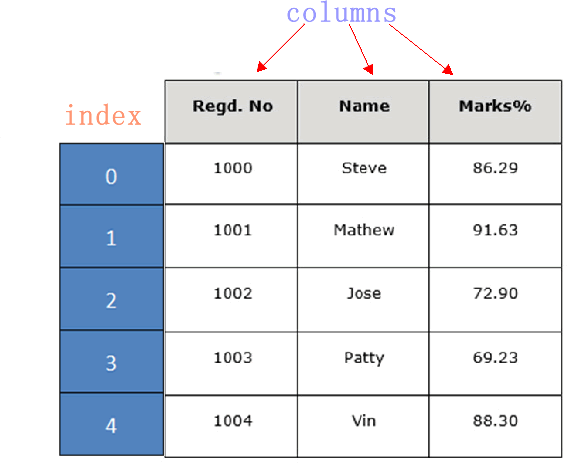DataFrame 结构类似于 Execl 的表格型，表格中列标签的含义如下所示：

• Regd.No：表示登记的序列号
• Name：学生姓名
• Marks：学生分数
• 同 Series 一样，DataFrame 自带行标签索引，默认为“隐式索引”即从 0 开始依次递增，行标签与 DataFrame 中的数据项一一对应。上述表格的行标签从 0 到 5，共记录了 5 条数据（图中将行标签省略）。当然你也可以用“显式索引”的方式来设置行标签。

下面对 DataFrame 数据结构的特点做简单地总结，如下所示：

• DataFrame 每一列的标签值允许使用不同的数据类型；
• DataFrame 是表格型的数据结构，具有行和列；
• DataFrame 中的每个数据值都可以被修改。
• DataFrame 结构的行数、列数允许增加或者删除；
• DataFrame 有两个方向的标签轴，分别是行标签和列标签；
• DataFrame 可以对行和列执行算术运算。
• ## 创建DataFrame对象

创建 DataFrame 对象的语法格式如下：

``````import pandas as pd
pd.DataFrame( data, index, columns, dtype, copy)
``````

参数说明：

参数名称 说明
data 输入的数据，可以是 ndarray，series，list，dict，标量以及一个 DataFrame。
index 行标签，如果没有传递 index 值，则默认行标签是 np.arange(n)，n 代表 data 的元素个数。
columns 列标签，如果没有传递 columns 值，则默认列标签是 np.arange(n)。
dtype dtype表示每一列的数据类型。
copy 默认为 False，表示复制数据 data。

Pandas 提供了多种创建 DataFrame 对象的方式，主要包含以下五种，分别进行介绍。

#### 1) 创建空的DataFrame对象

使用下列方式创建一个空的 DataFrame，这是 DataFrame 最基本的创建方法。

``````import pandas as pd
df = pd.DataFrame()
print(df)
``````

输出结果如下：

``````Empty DataFrame
Columns: []
Index: []
``````

#### 2) 列表创建DataFame对象

可以使用单一列表或嵌套列表来创建一个 DataFrame。

示例 1，单一列表创建 DataFrame：

``````import pandas as pd
data = [1,2,3,4,5]
df = pd.DataFrame(data)
print(df)
``````

输出如下：

``````     0
0    1
1    2
2    3
3    4
4    5
``````

示例 2，使用嵌套列表创建 DataFrame 对象：

``````import pandas as pd
data = [['Alex',10],['Bob',12],['Clarke',13]]
df = pd.DataFrame(data,columns=['Name','Age'])
print(df)
``````

输出结果：

``````      Name      Age
0     Alex      10
1     Bob       12
2     Clarke    13
``````

示例 3，指定数值元素的数据类型为 float：

``````import pandas as pd
data = [['Alex',10],['Bob',12],['Clarke',13]]
df = pd.DataFrame(data,columns=['Name','Age'],dtype=float)
print(df)
``````

输出结果：

``````      Name     Age
0     Alex     10.0
1     Bob      12.0
2     Clarke   13.0
``````

#### 3) 字典嵌套列表创建

data 字典中，键对应的值的元素长度必须相同（也就是列表长度相同）。如果传递了索引，那么索引的长度应该等于数组的长度；如果没有传递索引，那么默认情况下，索引将是 range(n)，其中 n 代表数组长度。

示例 4：

``````import pandas as pd
data = {'Name':['Tom', 'Jack', 'Steve', 'Ricky'],'Age':[28,34,29,42]}
df = pd.DataFrame(data)
print(df)
``````

输出结果：

``````      Age      Name
0     28        Tom
1     34       Jack
2     29      Steve
3     42      Ricky
``````

注意：这里使用了默认行标签，也就是 range(n)。它生成了 0,1,2,3，并分别对应了列表中的每个元素值。

示例 5，现在给上述示例 4 添加自定义的行标签：

``````import pandas as pd
data = {'Name':['Tom', 'Jack', 'Steve', 'Ricky'],'Age':[28,34,29,42]}
df = pd.DataFrame(data, index=['rank1','rank2','rank3','rank4'])
print(df)
``````

输出结果如下：

``````         Age    Name
rank1    28      Tom
rank2    34     Jack
rank3    29    Steve
rank4    42    Ricky
``````

注意：index 参数为每行分配了一个索引。

#### 4) 列表嵌套字典创建DataFrame对象

列表嵌套字典可以作为输入数据传递给 DataFrame 构造函数。默认情况下，字典的键被用作列名。

示例 6 如下：

``````import pandas as pd
data = [{'a': 1, 'b': 2},{'a': 5, 'b': 10, 'c': 20}]
df = pd.DataFrame(data)
print(df)
``````

输出结果：

``````    a    b      c
0   1   2     NaN
1   5   10   20.0
``````

注意：如果其中某个元素值缺失，也就是字典的 key 无法找到对应的 value，将使用 NaN 代替。

示例 7，给上述示例 6 添加行标签索引：

``````import pandas as pd
data = [{'a': 1, 'b': 2},{'a': 5, 'b': 10, 'c': 20}]
df = pd.DataFrame(data, index=['first', 'second'])
print(df)
``````

输出结果：

``````        a   b       c
first   1   2     NaN
second  5   10   20.0
``````

示例 8，如何使用字典嵌套列表以及行、列索引表创建一个 DataFrame 对象。

``````import pandas as pd
data = [{'a': 1, 'b': 2},{'a': 5, 'b': 10, 'c': 20}]
df1 = pd.DataFrame(data, index=['first', 'second'], columns=['a', 'b'])
df2 = pd.DataFrame(data, index=['first', 'second'], columns=['a', 'b1'])
print(df1)
print(df2)
``````

输出结果：

``````#df2输出
a  b
first    1  2
second   5  10

#df1输出
a  b1
first    1  NaN
second   5  NaN
``````

注意：因为 b1 在字典键中不存在，所以对应值为 NaN。

#### 5) Series创建DataFrame对象

您也可以传递一个字典形式的 Series，从而创建一个 DataFrame 对象，其输出结果的行索引是所有 index 的合集。 示例如下：

``````import pandas as pd
d = {'one' : pd.Series([1, 2, 3], index=['a', 'b', 'c']),
'two' : pd.Series([1, 2, 3, 4], index=['a', 'b', 'c', 'd'])}
df = pd.DataFrame(d)
print(df)
``````

输出结果如下：

``````      one    two
a     1.0    1
b     2.0    2
c     3.0    3
d     NaN    4
``````

注意：对于 one 列而言，此处虽然显示了行索引 ‘d’，但由于没有与其对应的值，所以它的值为 NaN。

## 列索引操作DataFrame

DataFrame 可以使用列索（columns index）引来完成数据的选取、添加和删除操作。下面依次对这些操作进行介绍。

#### 1) 列索引选取数据列

您可以使用列索引，轻松实现数据选取，示例如下：

``````import pandas as pd
d = {'one' : pd.Series([1, 2, 3], index=['a', 'b', 'c']),
'two' : pd.Series([1, 2, 3, 4], index=['a', 'b', 'c', 'd'])}
df = pd.DataFrame(d)
print(df ['one'])
``````

输出结果：

``````a     1.0
b     2.0
c     3.0
d     NaN
Name: one, dtype: float64
``````

#### 2) 列索引添加数据列

使用 columns 列索引表标签可以实现添加新的数据列，示例如下：

``````import pandas as pd
d = {'one' : pd.Series([1, 2, 3], index=['a', 'b', 'c']),
'two' : pd.Series([1, 2, 3, 4], index=['a', 'b', 'c', 'd'])}
df = pd.DataFrame(d)
#使用df['列']=值，插入新的数据列
df['three']=pd.Series([10,20,30],index=['a','b','c'])
print(df)
#将已经存在的数据列做相加运算
df['four']=df['one']+df['three']
print(df)
``````

输出结果：

``````使用列索引创建新数据列:
one   two   three
a    1.0    1    10.0
b    2.0    2    20.0
c    3.0    3    30.0
d    NaN    4    NaN

已存在的数据列做算术运算：
one   two   three    four
a     1.0    1    10.0     11.0
b     2.0    2    20.0     22.0
c     3.0    3    30.0     33.0
d     NaN    4     NaN     NaN
``````

上述示例，我们初次使用了 DataFrame 的算术运算，这和 NumPy 非常相似。除了使用`df[]=value`的方式外，您还可以使用 insert() 方法插入新的列，示例如下：

``````import pandas as pd
info=[['Jack',18],['Helen',19],['John',17]]
df=pd.DataFrame(info,columns=['name','age'])
print(df)
#注意是column参数
#数值1代表插入到columns列表的索引位置
df.insert(1,column='score',value=[91,90,75])
print(df)
``````

输出结果：

``````添加前：
name  age
0   Jack   18
1  Helen   19
2   John   17

添加后：
name  score  age
0   Jack     91   18
1  Helen     90   19
2   John     75   17
``````

#### 3) 列索引删除数据列

通过 del 和 pop() 都能够删除 DataFrame 中的数据列。示例如下：

``````import pandas as pd
d = {'one' : pd.Series([1, 2, 3], index=['a', 'b', 'c']),
'two' : pd.Series([1, 2, 3, 4], index=['a', 'b', 'c', 'd']),
'three' : pd.Series([10,20,30], index=['a','b','c'])}
df = pd.DataFrame(d)
print ("Our dataframe is:")
print(df)
#使用del删除
del df['one']
print(df)
#使用pop方法删除
df.pop('two')
print (df)
``````

输出结果：

``````原DataFrame:
one   three  two
a     1.0    10.0   1
b     2.0    20.0   2
c     3.0    30.0   3
d     NaN     NaN   4

使用del删除 first:
three    two
a     10.0     1
b     20.0     2
c     30.0     3
d     NaN      4

使用 pop()删除:
three
a  10.0
b  20.0
c  30.0
d  NaN
``````

## 行索引操作DataFrame

理解了上述的列索引操作后，行索引操作就变的简单。下面看一下，如何使用行索引来选取 DataFrame 中的数据。

#### 1) 标签索引选取

可以将行标签传递给 loc 函数，来选取数据。示例如下：

``````import pandas as pd
d = {'one' : pd.Series([1, 2, 3], index=['a', 'b', 'c']),
'two' : pd.Series([1, 2, 3, 4], index=['a', 'b', 'c', 'd'])}
df = pd.DataFrame(d)
print(df.loc['b'])
``````

输出结果：

``````one 2.0two 2.0Name: b, dtype: float64
``````

注意：loc 允许接两个参数分别是行和列，参数之间需要使用“逗号”隔开，但该函数只能接收标签索引。

#### 2) 整数索引选取

通过将数据行所在的索引位置传递给 iloc 函数，也可以实现数据行选取。示例如下：

``````import pandas as pd
d = {'one' : pd.Series([1, 2, 3], index=['a', 'b', 'c']),
'two' : pd.Series([1, 2, 3, 4], index=['a', 'b', 'c', 'd'])}
df = pd.DataFrame(d)
print (df.iloc）
``````

输出结果：

``````one   3.0
two   3.0
Name: c, dtype: float64
``````

注意：iloc 允许接受两个参数分别是行和列，参数之间使用“逗号”隔开，但该函数只能接收整数索引。

#### 3) 切片操作多行选取

您也可以使用切片的方式同时选取多行。示例如下：

``````import pandas as pd
d = {'one' : pd.Series([1, 2, 3], index=['a', 'b', 'c']),
'two' : pd.Series([1, 2, 3, 4], index=['a', 'b', 'c', 'd'])}
df = pd.DataFrame(d)
#左闭右开
print(df[2:4])
``````

输出结果：

``````   one  two
c  3.0    3
d  NaN    4
``````

#### 4) 添加数据行

使用 append() 函数，可以将新的数据行添加到 DataFrame 中，该函数会在行末追加数据行。示例如下：

``````import pandas as pd
df = pd.DataFrame([[1, 2], [3, 4]], columns = ['a','b'])
df2 = pd.DataFrame([[5, 6], [7, 8]], columns = ['a','b'])
#在行末追加新数据行
df = df.append(df2)
print(df)
``````

输出结果：

``````   a  b
0  1  2
1  3  4
0  5  6
1  7  8
``````

#### 5) 删除数据行

您可以使用行索引标签，从 DataFrame 中删除某一行数据。如果索引标签存在重复，那么它们将被一起删除。示例如下：

``````import pandas as pd
df = pd.DataFrame([[1, 2], [3, 4]], columns = ['a','b'])
df2 = pd.DataFrame([[5, 6], [7, 8]], columns = ['a','b'])
df = df.append(df2)
print(df)
#注意此处调用了drop()方法
df = df.drop(0)
print (df)
``````

输出结果：

``````执行drop(0)前：
a  b
0  1  2
1  3  4
0  5  6
1  7  8

执行drop(0)后：
a b
1 3 4
1 7 8
``````

在上述的示例中，默认使用 range(2) 生成了行索引，并通过 drop(0) 同时删除了两行数据。

## 常用属性和方法汇总

DataFrame 的属性和方法，与 Series 相差无几，如下所示：

名称 属性&方法描述
T 行和列转置。
axes 返回一个仅以行轴标签和列轴标签为成员的列表。
dtypes 返回每列数据的数据类型。
empty DataFrame中没有数据或者任意坐标轴的长度为0，则返回True。
ndim 轴的数量，也指数组的维数。
shape 返回一个元组，表示了 DataFrame 维度。
size DataFrame中的元素数量。
values 使用 numpy 数组表示 DataFrame 中的元素值。
tail() 返回后 n 行数据。
shift() 将行或列移动指定的步幅长度

下面对 DataFrame 常用属性进行演示，首先我们创建一个 DataFrame 对象，示例如下：

``````import pandas as pd
import numpy as np
d = {'Name':pd.Series(['c语言中文网','编程帮',"百度",'360搜索','谷歌','微学苑','Bing搜索']),
'years':pd.Series([5,6,15,28,3,19,23]),
'Rating':pd.Series([4.23,3.24,3.98,2.56,3.20,4.6,3.8])}
#构建DataFrame
df = pd.DataFrame(d)
#输出series
print(df)
``````

输出结果：

``````输出 series 数据:
Name  years  Rating
0  c语言中文网    5    4.23
1     编程帮     6    3.24
2      百度     15    3.98
3   360搜索     28    2.56
4      谷歌     3     3.20
5     微学苑    19    4.60
6  Bing搜索     23    3.80
``````

#### 1) T（Transpose）转置

返回 DataFrame 的转置，也就是把行和列进行交换。

``````import pandas as pd
import numpy as np
d = {'Name':pd.Series(['c语言中文网','编程帮',"百度",'360搜索','谷歌','微学苑','Bing搜索']),
'years':pd.Series([5,6,15,28,3,19,23]),
'Rating':pd.Series([4.23,3.24,3.98,2.56,3.20,4.6,3.8])}
#构建DataFrame
df = pd.DataFrame(d)
#输出DataFrame的转置
print(df.T)
``````

输出结果：

``````Our data series is:
0         1      2      3       4    5       6
Name    c语言中文网   编程帮    百度  360搜索   谷歌  微学苑  Bing搜索
years        5        6      15      28      3     19      23
Rating    4.23       3.24    3.98    2.56    3.2   4.6     3.8
``````

#### 2) axes

返回一个行标签、列标签组成的列表。

``````import pandas as pd
import numpy as np
d = {'Name':pd.Series(['c语言中文网','编程帮',"百度",'360搜索','谷歌','微学苑','Bing搜索']),
'years':pd.Series([5,6,15,28,3,19,23]),
'Rating':pd.Series([4.23,3.24,3.98,2.56,3.20,4.6,3.8])}
#构建DataFrame
df = pd.DataFrame(d)
#输出行、列标签
print(df.axes)
``````

输出结果：

``````[RangeIndex(start=0, stop=7, step=1), Index(['Name', 'years', 'Rating'], dtype='object')]
``````

#### 3) dtypes

返回每一列的数据类型。示例如下：

``````import pandas as pd
import numpy as np
d = {'Name':pd.Series(['c语言中文网','编程帮',"百度",'360搜索','谷歌','微学苑','Bing搜索']),
'years':pd.Series([5,6,15,28,3,19,23]),
'Rating':pd.Series([4.23,3.24,3.98,2.56,3.20,4.6,3.8])}
#构建DataFrame
df = pd.DataFrame(d)
#输出行、列标签
print(df.dtypes)
``````

输出结果：

``````Name       object
years       int64
Rating     float64
dtype:     object
``````

#### 4) empty

返回一个布尔值，判断输出的数据对象是否为空，若为 True 表示对象为空。

``````import pandas as pd
import numpy as np
d = {'Name':pd.Series(['c语言中文网','编程帮',"百度",'360搜索','谷歌','微学苑','Bing搜索']),
'years':pd.Series([5,6,15,28,3,19,23]),
'Rating':pd.Series([4.23,3.24,3.98,2.56,3.20,4.6,3.8])}
#构建DataFrame
df = pd.DataFrame(d)
#判断输入数据是否为空
print(df.empty)
``````

输出结果：

``````判断输入对象是否为空：
False
``````

#### 5) ndim

返回数据对象的维数。DataFrame 是一个二维数据结构。

``````import pandas as pd
import numpy as np
d = {'Name':pd.Series(['c语言中文网','编程帮',"百度",'360搜索','谷歌','微学苑','Bing搜索']),
'years':pd.Series([5,6,15,28,3,19,23]),
'Rating':pd.Series([4.23,3.24,3.98,2.56,3.20,4.6,3.8])}
#构建DataFrame
df = pd.DataFrame(d)
#DataFrame的维度
print(df.ndim)
``````

输出结果：

2

#### 6) shape

返回一个代表 DataFrame 维度的元组。返回值元组 (a,b)，其中 a 表示行数，b 表示列数。

``````import pandas as pd
import numpy as np
d = {'Name':pd.Series(['c语言中文网','编程帮',"百度",'360搜索','谷歌','微学苑','Bing搜索']),
'years':pd.Series([5,6,15,28,3,19,23]),
'Rating':pd.Series([4.23,3.24,3.98,2.56,3.20,4.6,3.8])}
#构建DataFrame
df = pd.DataFrame(d)
#DataFrame的形状
print(df.shape)
输出结果：
``````

(7, 3)

#### 7) size

返回 DataFrame 中的元素数量。示例如下：

``````import pandas as pd
import numpy as np
d = {'Name':pd.Series(['c语言中文网','编程帮',"百度",'360搜索','谷歌','微学苑','Bing搜索']),
'years':pd.Series([5,6,15,28,3,19,23]),
'Rating':pd.Series([4.23,3.24,3.98,2.56,3.20,4.6,3.8])}
#构建DataFrame
df = pd.DataFrame(d)
#DataFrame的中元素个数
print(df.size)
``````

输出结果：

21

#### 8) values

以 ndarray 数组的形式返回 DataFrame 中的数据。

``````import pandas as pd
import numpy as np
d = {'Name':pd.Series(['c语言中文网','编程帮',"百度",'360搜索','谷歌','微学苑','Bing搜索']),
'years':pd.Series([5,6,15,28,3,19,23]),
'Rating':pd.Series([4.23,3.24,3.98,2.56,3.20,4.6,3.8])}
#构建DataFrame
df = pd.DataFrame(d)
#DataFrame的数据
print(df.values)
``````

输出结果：

``````[['c语言中文网' 5 4.23]
['编程帮' 6 3.24]
['百度' 15 3.98]
['360搜索' 28 2.56]
['谷歌' 3 3.2]
['微学苑' 19 4.6]
['Bing搜索' 23 3.8]]
``````

如果想要查看 DataFrame 的一部分数据，可以使用 head() 或者 tail() 方法。其中 head() 返回前 n 行数据，默认显示前 5 行数据。示例如下：

``````import pandas as pd
import numpy as np
d = {'Name':pd.Series(['c语言中文网','编程帮',"百度",'360搜索','谷歌','微学苑','Bing搜索']),
'years':pd.Series([5,6,15,28,3,19,23]),
'Rating':pd.Series([4.23,3.24,3.98,2.56,3.20,4.6,3.8])}
#构建DataFrame
df = pd.DataFrame(d)
#获取前3行数据
``````

输出结果：

``````     Name       years   Rating
0   c语言中文网      5     4.23
1    编程帮         6     3.24
2    百度          15     3.98
``````

tail() 返回后 n 行数据，示例如下：

``````import pandas as pd
import numpy as np
d = {'Name':pd.Series(['c语言中文网','编程帮',"百度",'360搜索','谷歌','微学苑','Bing搜索']),
'years':pd.Series([5,6,15,28,3,19,23]),
'Rating':pd.Series([4.23,3.24,3.98,2.56,3.20,4.6,3.8])}
#构建DataFrame
df = pd.DataFrame(d)
#获取后2行数据
print(df.tail(2))
``````

输出结果：

``````      Name     years   Rating
5     微学苑      19     4.6
6    Bing搜索     23     3.8
``````

#### 10) shift()移动行或列

如果您想要移动 DataFrame 中的某一行/列，可以使用 shift() 函数实现。它提供了一个`periods`参数，该参数表示在特定的轴上移动指定的步幅。

shif() 函数的语法格式如下：

DataFrame.shift(periods=1, freq=None, axis=0)

参数说明如下：

参数名称 说明
peroids 类型为int，表示移动的幅度，可以是正数，也可以是负数，默认值为1。
freq 日期偏移量，默认值为None，适用于时间序。取值为符合时间规则的字符串。
axis 如果是 0 或者 “index” 表示上下移动，如果是 1 或者 “columns” 则会左右移动。
fill_value 该参数用来填充缺失值。

该函数的返回值是移动后的 DataFrame 副本。下面看一组简单的实例：

``````import pandas as pd
info= pd.DataFrame({'a_data': [40, 28, 39, 32, 18],
'b_data': [20, 37, 41, 35, 45],
'c_data': [22, 17, 11, 25, 15]})
#移动幅度为3
info.shift(periods=3)
``````

输出结果：

``````   a_data  b_data  c_data
0     NaN     NaN     NaN
1     NaN     NaN     NaN
2     NaN     NaN     NaN
3    40.0    20.0    22.0
4    28.0    37.0    17.0
``````

下面使用 fill_value 参数填充 DataFrame 中的缺失值，如下所示：

``````import pandas as pd
info= pd.DataFrame({'a_data': [40, 28, 39, 32, 18],
'b_data': [20, 37, 41, 35, 45],
'c_data': [22, 17, 11, 25, 15]})
#移动幅度为3
print(info.shift(periods=3))
#将缺失值和原数值替换为52
info.shift(periods=3,axis=1,fill_value= 52)
``````

输出结果：

``````原输出结果：
a_data  b_data  c_data
0     NaN     NaN     NaN
1     NaN     NaN     NaN
2     NaN     NaN     NaN
3    40.0    20.0    22.0
4    28.0    37.0    17.0

替换后输出：
a_data  b_data  c_data
0      52      52      52
1      52      52      52
2      52      52      52
3      52      52      52
4      52      52      52
``````

注意：fill_value 参数不仅可以填充缺失值，还也可以对原数据进行替换。

# Pandas Panel三维数据结构

Panel 结构也称“面板结构”，它源自于 Panel Data 一词，翻译为“面板数据”。如果您使用的是 Pandas 0.25 以前的版本，那么您需要掌握本节内容，否则，作为了解内容即可。

自 Pandas 0.25 版本后， Panel 结构已经被废弃。

Panel 是一个用来承载数据的三维数据结构，它有三个轴，分别是 items（0 轴），major_axis（1 轴），而 minor_axis（2 轴）。这三个轴为描述、操作 Panel 提供了支持，其作用介绍如下：

• items：axis =0，Panel 中的每个 items 都对应一个 DataFrame。
• major_axis：axis=1，用来描述每个 DataFrame 的行索引。
• minor_axis：axis=2，用来描述每个 DataFrame 的列索引。
• ## pandas.Panel()

您可以使用下列构造函数创建一个 Panel，如下所示：

pandas.Panel(data, items, major_axis, minor_axis, dtype, copy)

参数说明如下：

参数名称 描述说明
data 输入数据，可以是 ndarray，Series，列表，字典，或者 DataFrame。
items axis=0
major_axis axis=1
minor_axis axis=2
dtype 每一列的数据类型。
copy 默认为 False，表示是否复制数据。

## 创建Panel 对象

下面介绍创建 Panel 对象的两种方式：一种是使用 nadarry 数组创建，另一种使用 DataFrame 对象创建。首先，我们学习如何创建一个空的 Panel 对象。

#### 1) 创建一个空Panel

使用 Panel 的构造函数创建，如下所示：

``````import pandas as pd
p = pd.Panel()
print(p)
``````

输出结果：

``````<class 'pandas.core.panel.Panel'>
Dimensions: 0 (items) x 0 (major_axis) x 0 (minor_axis)
Items axis: None
Major_axis axis: None
Minor_axis axis: None
``````

#### 2) ndarray三维数组创建

``````import pandas as pd
import numpy as np
#返回均匀分布的随机样本值位于[0,1）之间
data = np.random.rand(2,4,5)
p = pd.Panel(data)
print (p)
``````

输出结果：

``````<class 'pandas.core.panel.Panel'>
Dimensions: 2 (items) x 4 (major_axis) x 5 (minor_axis)
Items axis: 0 to 1
Major_axis axis: 0 to 3
Minor_axis axis: 0 to 4
``````

请注意与上述示例的空 Panel 进行对比。

#### 3) DataFrame创建

下面使用 DataFrame 创建一个 Panel，示例如下：

``````import pandas as pd
import numpy as np
data = {'Item1' : pd.DataFrame(np.random.randn(4, 3)),
'Item2' : pd.DataFrame(np.random.randn(4, 2))}
p = pd.Panel(data)
print(p)
``````

输出结果：

``````Dimensions: 2 (items) x 4 (major_axis) x 3 (minor_axis)
Items axis: Item1 to Item2
Major_axis axis: 0 to 3
Minor_axis axis: 0 to 2
``````

## Panel中选取数据

如果想要从 Panel 对象中选取数据，可以使用 Panel 的三个轴来实现，也就是`items``major_axis``minor_axis`。下面介绍其中一种，大家体验一下即可。

#### 1) 使用 items选取数据

示例如下：

``````import pandas as pd
import numpy as np
data = {'Item1':pd.DataFrame(np.random.randn(4, 3)),
'Item2':pd.DataFrame(np.random.randn(4, 2))}
p = pd.Panel(data)
print(p['Item1'])
``````

输出结果：

``````            0          1          2
0    0.488224  -0.128637   0.930817
1    0.417497   0.896681   0.576657
2   -2.775266   0.571668   0.290082
3   -0.400538  -0.144234   1.110535
``````

上述示例中 data，包含了两个数据项，我们选择了 item1，输出结果是 4 行 3 列的 DataFrame，其行、列索引分别对应 major_axis 和 minor_axis。

# Python Pandas描述性统计

描述统计学（descriptive statistics）是一门统计学领域的学科，主要研究如何取得反映客观现象的数据，并以图表形式对所搜集的数据进行处理和显示，最终对数据的规律、特征做出综合性的描述分析。Pandas 库正是对描述统计学知识完美应用的体现，可以说如果没有“描述统计学”作为理论基奠，那么 Pandas 是否存在犹未可知。下列表格对 Pandas 常用的统计学函数做了简单的总结：

函数名称 描述说明
count() 统计某个非空值的数量。
sum() 求和
mean() 求均值
median() 求中位数
mode() 求众数
std() 求标准差
min() 求最小值
max() 求最大值
abs() 求绝对值
prod() 求所有数值的乘积。
cumsum() 计算累计和，axis=0，按照行累加；axis=1，按照列累加。
cumprod() 计算累计积，axis=0，按照行累积；axis=1，按照列累积。
corr() 计算数列或变量之间的相关系数，取值-1到1，值越大表示关联性越强。

从描述统计学角度出发，我们可以对 DataFrame 结构执行聚合计算等其他操作，比如 sum() 求和、mean()求均值等方法。

在 DataFrame 中，使用聚合类方法时需要指定轴(axis)参数。下面介绍两种传参方式：

• 对行操作，默认使用 axis=0 或者使用 “index”；
• 对列操作，默认使用 axis=1 或者使用 “columns”。
•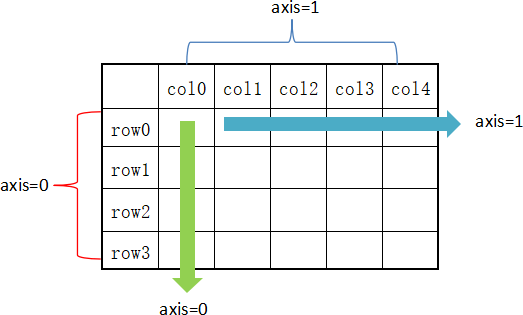图1：axis轴示意图

从图 1 可以看出，axis=0 表示按垂直方向进行计算，而 axis=1 则表示按水平方向。下面让我们创建一个 DataFrame，使用它对本节的内容进行演示。

创建一个 DataFrame 结构，如下所示：

``````import pandas as pd
import numpy as np
#创建字典型series结构
d = {'Name':pd.Series(['小明','小亮','小红','小华','老赵','小曹','小陈',
'老李','老王','小冯','小何','老张']),
'Age':pd.Series([25,26,25,23,30,29,23,34,40,30,51,46]),
'Rating':pd.Series([4.23,3.24,3.98,2.56,3.20,4.6,3.8,3.78,2.98,4.80,4.10,3.65])
}
df = pd.DataFrame(d)
print(df)
``````

输出结果：

``````   Name  Age  Rating
0    小明   25    4.23
1    小亮   26    3.24
2    小红   25    3.98
3    小华   23    2.56
4    老赵   30    3.20
5    小曹   29    4.60
6    小陈   23    3.80
7    老李   34    3.78
8    老王   40    2.98
9    小冯   30    4.80
10   小何   51    4.10
11   老张   46    3.65
``````

## sum()求和

在默认情况下，返回 axis=0 的所有值的和。示例如下：

``````import pandas as pd
import numpy as np
#创建字典型series结构
d = {'Name':pd.Series(['小明','小亮','小红','小华','老赵','小曹','小陈',
'老李','老王','小冯','小何','老张']),
'Age':pd.Series([25,26,25,23,30,29,23,34,40,30,51,46]),
'Rating':pd.Series([4.23,3.24,3.98,2.56,3.20,4.6,3.8,3.78,2.98,4.80,4.10,3.65])
}
df = pd.DataFrame(d)
#默认axis=0或者使用sum("index")
print(df.sum())
``````

输出结果：

``````Name      小明小亮小红小华老赵小曹小陈老李老王小冯小何老张
Age                            382
Rating                       44.92
dtype: object
``````

注意：sum() 和 cumsum() 函数可以同时处理数字和字符串数据。虽然字符聚合通常不被使用，但使用这两个函数并不会抛出异常；而对于 abs()、cumprod() 函数则会抛出异常，因为它们无法操作字符串数据。

下面再看一下 axis=1 的情况，如下所示：

``````import pandas as pd
import numpy as np
d = {'Name':pd.Series(['小明','小亮','小红','小华','老赵','小曹','小陈',
'老李','老王','小冯','小何','老张']),
'Age':pd.Series([25,26,25,23,30,29,23,34,40,30,51,46]),
'Rating':pd.Series([4.23,3.24,3.98,2.56,3.20,4.6,3.8,3.78,2.98,4.80,4.10,3.65])
}
df = pd.DataFrame(d)
#也可使用sum("columns")或sum(1)
print(df.sum(axis=1))
``````

输出结果：

``````0     29.23
1     29.24
2     28.98
3     25.56
4     33.20
5     33.60
6     26.80
7     37.78
8     42.98
9     34.80
10    55.10
11    49.65
dtype: float64
``````

## mean()求均值

示例如下：

``````import pandas as pd
import numpy as np
d = {'Name':pd.Series(['小明','小亮','小红','小华','老赵','小曹','小陈',
'老李','老王','小冯','小何','老张']),
'Age':pd.Series([25,26,25,23,30,29,23,34,40,30,51,46]),
'Rating':pd.Series([4.23,3.24,3.98,2.56,3.20,4.6,3.8,3.78,2.98,4.80,4.10,3.65])
}
df = pd.DataFrame(d)
print(df.mean())
``````

输出结果：

``````Age       31.833333
Rating     3.743333
dtype: float64
``````

## std()求标准差

返回数值列的标准差，示例如下：

``````import pandas as pd
import numpy as np
d = {'Name':pd.Series(['小明','小亮','小红','小华','老赵','小曹','小陈',
'老李','老王','小冯','小何','老张']),
'Age':pd.Series([25,26,25,23,59,19,23,44,40,30,51,54]),
'Rating':pd.Series([4.23,3.24,3.98,2.56,3.20,4.6,3.8,3.78,2.98,4.80,4.10,3.65])
}
df = pd.DataFrame(d)
print(df.std())
``````

输出结果：

``````Age       13.976983
Rating     0.661628
dtype: float64
``````

标准差是方差的算术平方根，它能反映一个数据集的离散程度。注意，平均数相同的两组数据，标准差未必相同。

## 数据汇总描述

describe() 函数显示与 DataFrame 数据列相关的统计信息摘要。示例如下：

``````import pandas as pd
import numpy as np
d = {'Name':pd.Series(['小明','小亮','小红','小华','老赵','小曹','小陈',
'老李','老王','小冯','小何','老张']),
'Age':pd.Series([25,26,25,23,30,29,23,34,40,30,51,46]),
'Rating':pd.Series([4.23,3.24,3.98,2.56,3.20,4.6,3.8,3.78,2.98,4.80,4.10,3.65])
}
#创建DataFrame对象
df = pd.DataFrame(d)
#求出数据的所有描述信息
print(df.describe())
``````

输出结果：

``````             Age     Rating
count  12.000000  12.000000
mean   34.916667   3.743333
std    13.976983   0.661628
min    19.000000   2.560000
25%    24.500000   3.230000
50%    28.000000   3.790000
75%    45.750000   4.132500
max    59.000000   4.800000
``````

describe() 函数输出了平均值、std 和 IQR 值(四分位距)等一系列统计信息。通过 describe() 提供的`include`能够筛选字符列或者数字列的摘要信息。

include 相关参数值说明如下：

• object： 表示对字符列进行统计信息描述；
• number：表示对数字列进行统计信息描述；
• all：汇总所有列的统计信息。
• 下面看一组示例，如下所示：

``````import pandas as pd
import numpy as np
d = {'Name':pd.Series(['小明','小亮','小红','小华','老赵','小曹','小陈',
'老李','老王','小冯','小何','老张']),
'Age':pd.Series([25,26,25,23,59,19,23,44,40,30,51,54]),
'Rating':pd.Series([4.23,3.24,3.98,2.56,3.20,4.6,3.8,3.78,2.98,4.80,4.10,3.65])
}
df = pd.DataFrame(d)
print(df.describe(include=["object"]))
``````

输出结果：

``````       Name
count    12
unique   12
top      小红
freq      1
``````

最后使用`all`参数，看一下输出结果，如下所示：

``````import pandas as pd
import numpy as np
d = {'Name':pd.Series(['小明','小亮','小红','小华','老赵','小曹','小陈',
'老李','老王','小冯','小何','老张']),
'Age':pd.Series([25,26,25,23,59,19,23,44,40,30,51,54]),
'Rating':pd.Series([4.23,3.24,3.98,2.56,3.20,4.6,3.8,3.78,2.98,4.80,4.10,3.65])
}
df = pd.DataFrame(d)
print(df.describe(include="all"))
``````

输出结果：

``````       Name        Age     Rating
count    12  12.000000  12.000000
unique   12        NaN        NaN
top      小红       NaN       NaN
freq      1        NaN        NaN
mean    NaN  34.916667   3.743333
std     NaN  13.976983   0.661628
min     NaN  19.000000   2.560000
25%     NaN  24.500000   3.230000
50%     NaN  28.000000   3.790000
75%     NaN  45.750000   4.132500
max     NaN  59.000000   4.800000
``````

# Python Pandas绘图教程（详解版）

Pandas 在数据分析、数据可视化方面有着较为广泛的应用，Pandas 对 Matplotlib 绘图软件包的基础上单独封装了一个`plot()`接口，通过调用该接口可以实现常用的绘图操作。本节我们深入讲解一下 Pandas 的绘图操作。

Pandas 之所以能够实现了数据可视化，主要利用了 Matplotlib 库的 plot() 方法，它对 plot() 方法做了简单的封装，因此您可以直接调用该接口。下面看一组简单的示例：

``````import pandas as pd
import numpy as np
#创建包含时间序列的数据
df = pd.DataFrame(np.random.randn(8,4),index=pd.date_range('2/1/2020',periods=8), columns=list('ABCD'))
df.plot()
``````

输结果图，如下所示：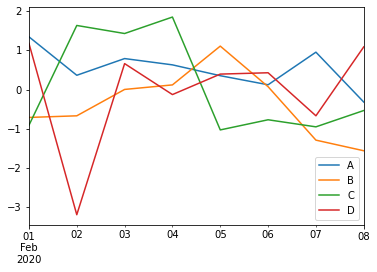图1：Pandas绘图

如上图所示，如果行索引中包含日期，Pandas 会自动调用 gct().autofmt_xdate() 来格式化 x 轴。

除了使用默认的线条绘图外，您还可以使用其他绘图方式，如下所示：

• 柱状图：bar() 或 barh()
• 直方图：hist()
• 箱状箱：box()
• 区域图：area()
• 散点图：scatter()
• 通过关键字参数`kind`可以把上述方法传递给 plot()。

## 柱状图

创建一个柱状图，如下所示：

``````import pandas as pd
import numpy as np
df = pd.DataFrame(np.random.rand(10,4),columns=['a','b','c','d','e'])
#或使用df.plot(kind="bar")
df.plot.bar()
``````

输出结果：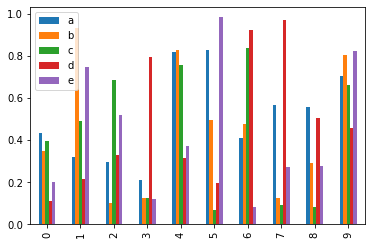图2：Pandas绘制柱状图

通过设置参数`stacked=True`可以生成柱状堆叠图，示例如下：

``````import pandas as pd
import numpy as np
df = pd.DataFrame(np.random.rand(10,5),columns=['a','b','c','d','e'])
df.plot(kind="bar",stacked=True)
#或者使用df.plot.bar(stacked="True")
``````

输出结果：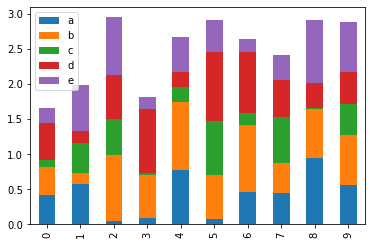图3：Pandas绘制柱状图

如果要绘制水平柱状图，您可以使用以下方法：

``````import pandas as pd
import numpy as np
df = pd.DataFrame(np.random.rand(10,4),columns=['a','b','c','d'])
print(df)
df.plot.barh(stacked=True)
``````

输出结果：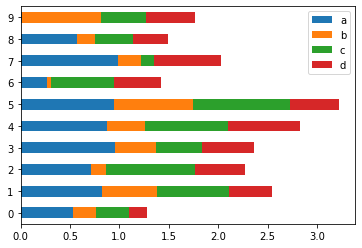图4：水平柱状图

## 直方图

plot.hist() 可以实现绘制直方图，并且它还可以指定 bins（构成直方图的箱数）。

``````import pandas as pd
import numpy as np
df = pd.DataFrame({'A':np.random.randn(100)+2,'B':np.random.randn(100),'C':
np.random.randn(100)-2}, columns=['A', 'B', 'C'])
print(df)
#指定箱数为15
df.plot.hist(bins=15)
``````

输出结果：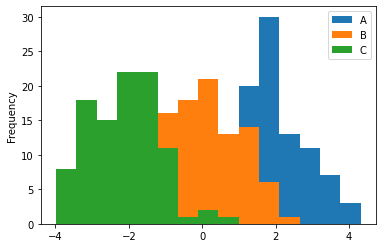图5：绘制直方图

给每一列数据都绘制一个直方图，需要使以下方法：

``````import pandas as pd
import numpy as np
df = pd.DataFrame({'A':np.random.randn(100)+2,'B':np.random.randn(100),'C':
np.random.randn(100)-2,'D':np.random.randn(100)+3},columns=['A', 'B', 'C','D'])
#使用diff绘制
df.diff().hist(color="r",alpha=0.5,bins=15)
``````

输出结果：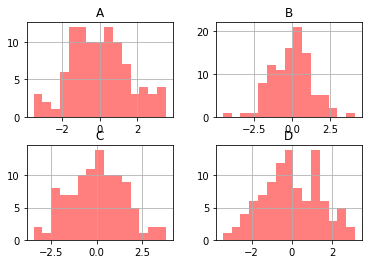图6：直方图绘制

## 箱型图

通过调用 Series.box.plot() 、DataFrame.box.plot() 或者 DataFrame.boxplot() 方法来绘制箱型图，它将每一列数据的分布情况，以可视化的图像展现出来。

``````import pandas as pd
import numpy as np
df = pd.DataFrame(np.random.rand(10, 4), columns=['A', 'B', 'C', 'D'])
df.plot.box()
``````

输出结果：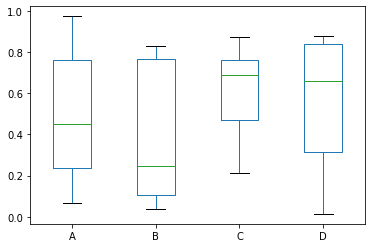图7：绘制箱型图

## 区域图

您可以使用 Series.plot.area() 或 DataFrame.plot.area() 方法来绘制区域图。

``````import pandas as pd
import numpy as np
df = pd.DataFrame(np.random.rand(5, 4), columns=['a', 'b', 'c', 'd'])
df.plot.area()
``````

输出结果：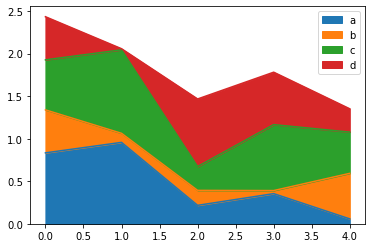图8：绘制区域图

## 散点图

使用 DataFrame.plot.scatter() 方法来绘制散点图，如下所示：

``````import pandas as pd
import numpy as np
df = pd.DataFrame(np.random.rand(30, 4), columns=['a', 'b', 'c', 'd'])
df.plot.scatter(x='a',y='b')
``````

输出结果：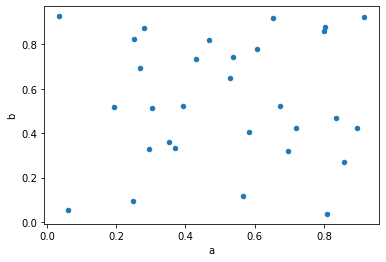图9：绘制散点图

## 饼状图

饼状图可以通过 DataFrame.plot.pie() 方法来绘制。示例如下：

``````import pandas as pd
import numpy as np
df = pd.DataFrame(3 * np.random.rand(4), index=['go', 'java', 'c++', 'c'], columns=['L'])
df.plot.pie(subplots=True)
``````

输出结果：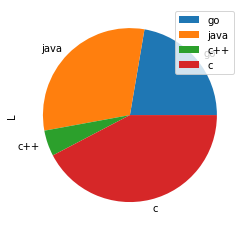图10：Pandas绘制饼状图

# Pandas csv读写文件

在《Python Pandas读取文件》中，我们讲解了多种用 Pandas 读写文件的方法。本节我们讲解如何应用这些方法 。

``````pandas.read_csv(filepath_or_buffer, sep=',', delimiter=None, header='infer',names=None, index_col=None, usecols=None)
``````

下面，新建一个 txt 文件，并添加以下数据：

``````ID,Name,Age,City,Salary
1,Jack,28,Beijing,22000
2,Lida,32,Shanghai,19000
3,John,43,Shenzhen,12000
4,Helen,38,Hengshui,3500
``````

将 txt 文件另存为 person.csv 文件格式，直接修改文件扩展名即可。接下来，对此文件进行操作。

read_csv() 表示从 CSV 文件中读取数据，并创建 DataFrame 对象。

``````import pandas as pd
#需要注意文件的路径
print (df)
``````

输出结果：

``````   ID   Name  Age      City  Salary
0   1   Jack   28   Beijing   22000
1   2   Lida   32  Shanghai   19000
2   3   John   43  Shenzhen   12000
3   4  Helen   38  Hengshui    3500
``````

#### 1) 自定义索引

在 CSV 文件中指定了一个列，然后使用`index_col`可以实现自定义索引。

``````import pandas as pd
print(df)
``````

输出结果：

``````     Name  Age      City  Salary
ID
1    Jack   28   Beijing   22000
2    Lida   32  Shanghai   19000
3    John   43  Shenzhen   12000
4   Helen   38  Hengshui    3500
``````

#### 2) 查看每一列的dtype

``````import pandas as pd
#转换salary为float类型
print(df.dtypes)
``````

输出结果：

``````ID          int64
Name       object
Age         int64
City       object
Salary    float64
dtype: object
``````

注意：默认情况下，Salary 列的 dtype 是 int 类型，但结果显示其为 float 类型，因为我们已经在上述代码中做了类型转换。

#### 3) 更改文件标头名

使用 names 参数可以指定头文件的名称。

``````import pandas as pd
print(df)
``````

输出结果：

``````    a      b    c         d       e
0  ID   Name  Age      City  Salary
1   1   Jack   28   Beijing   22000
2   2   Lida   32  Shanghai   19000
3   3   John   43  Shenzhen   12000
4   4  Helen   38  Hengshui    3500
``````

注意：文件标头名是附加的自定义名称，但是您会发现，原来的标头名（列标签名）并没有被删除，此时您可以使用`header`参数来删除它。

通过传递标头所在行号实现删除，如下所示：

``````import pandas as pd
print(df)
``````

输出结果：

``````   a      b   c         d      e
0  1   Jack  28   Beijing  22000
1  2   Lida  32  Shanghai  19000
2  3   John  43  Shenzhen  12000
3  4  Helen  38  Hengshui   3500
``````

假如原标头名并没有定义在第一行，您也可以传递相应的行号来删除它。

#### 4) 跳过指定的行数

`skiprows`参数表示跳过指定的行数。

``````import pandas as pd
print(df)
``````

输出结果：

``````   2   Lida  32  Shanghai  19000
0  3   John  43  Shenzhen  12000
1  4  Helen  38  Hengshui   3500
``````

注意：包含标头所在行。

## to_csv()

Pandas 提供的 to_csv() 函数用于将 DataFrame 转换为 CSV 数据。如果想要把 CSV 数据写入文件，只需向函数传递一个文件对象即可。否则，CSV 数据将以字符串格式返回。

下面看一组简单的示例：

``````import pandas as pd
data = {'Name': ['Smith', 'Parker'], 'ID': [101, 102], 'Language': ['Python', 'JavaScript']}
info = pd.DataFrame(data)
print('DataFrame Values:\n', info)
#转换为csv数据
csv_data = info.to_csv()
print('\nCSV String Values:\n', csv_data)
``````

输出结果：

``````DataFrame:
Name   ID    Language
0   Smith  101      Python
1  Parker  102  JavaScript

csv数据:
,Name,ID,Language
0,Smith,101,Python
1,Parker,102,JavaScript
``````

指定 CSV 文件输出时的分隔符，并将其保存在 pandas.csv 文件中，代码如下：

``````import pandas as pd
#注意：pd.NaT表示null缺失数据
data = {'Name': ['Smith', 'Parker'], 'ID': [101, pd.NaT], 'Language': ['Python', 'JavaScript']}
info = pd.DataFrame(data)
``````

# Pandas Excel读写操作详解

Excel 是由微软公司开发的办公软件之一，它在日常工作中得到了广泛的应用。在数据量较少的情况下，Excel 对于数据的处理、分析、可视化有其独特的优势，因此可以显著提升您的工作效率。但是，当数据量非常大时，Excel 的劣势就暴露出来了，比如，操作重复、数据分析难等问题。Pandas 提供了操作 Excel 文件的函数，可以很方便地处理 Excel 表格。

## to_excel()

通过 to_excel() 函数可以将 Dataframe 中的数据写入到 Excel 文件。

如果想要把单个对象写入 Excel 文件，那么必须指定目标文件名；如果想要写入到多张工作表中，则需要创建一个带有目标文件名的`ExcelWriter`对象，并通过`sheet_name`参数依次指定工作表的名称。

to_ecxel() 语法格式如下：

``````DataFrame.to_excel(excel_writer, sheet_name='Sheet1', na_rep='', float_format=None, columns=None, header=True, index=True, index_label=None, startrow=0, startcol=0, engine=None, merge_cells=True, encoding=None, inf_rep='inf', verbose=True, freeze_panes=None)
``````

下表列出函数的常用参数项，如下表所示：

参数名称 描述说明
excel_wirter 文件路径或者 ExcelWrite 对象。
sheet_name 指定要写入数据的工作表名称。
na_rep 缺失值的表示形式。
float_format 它是一个可选参数，用于格式化浮点数字符串。
columns 指要写入的列。
index 表示要写入的索引。
index_label 引用索引列的列标签。如果未指定，并且 hearder 和 index 均为为 True，则使用索引名称。如果 DataFrame 使用 MultiIndex，则需要给出一个序列。
startrow 初始写入的行位置，默认值0。表示引用左上角的行单元格来储存 DataFrame。
startcol 初始写入的列位置，默认值0。表示引用左上角的列单元格来储存 DataFrame。
engine 它是一个可选参数，用于指定要使用的引擎，可以是 openpyxl 或 xlsxwriter。

下面看一组简单的示例：

``````import pandas as pd
#创建DataFrame数据
info_website = pd.DataFrame({'name': ['编程帮', 'c语言中文网', '微学苑', '92python'],
'rank': [1, 2, 3, 4],
'language': ['PHP', 'C', 'PHP','Python' ],
'url': ['www.bianchneg.com', 'c.bianchneg.net', 'www.weixueyuan.com','www.92python.com' ]})
#创建ExcelWrite对象
writer = pd.ExcelWriter('website.xlsx')
info_website.to_excel(writer)
writer.save()
print('输出成功')
``````

上述代码执行后会自动生成 website.xlsx 文件，文件内容如下：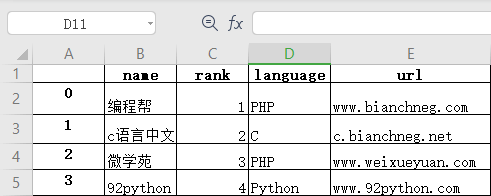图1：DataFrame转为Excel

``````pd.read_excel(io, sheet_name=0, header=0, names=None, index_col=None,
usecols=None, squeeze=False,dtype=None, engine=None,
converters=None, true_values=None, false_values=None,
skiprows=None, nrows=None, na_values=None, parse_dates=False,
date_parser=None, thousands=None, comment=None, skipfooter=0,
convert_float=True, **kwds)
``````

下表对常用参数做了说明：

参数名称 说明
io 表示 Excel 文件的存储路径。
sheet_name 要读取的工作表名称。
names 一般适用于Excel缺少列名，或者需要重新定义列名的情况；names的长度必须等于Excel表格列的长度，否则会报错。
index_col 用做行索引的列，可以是工作表的列名称，如 index_col = ‘列名’，也可以是整数或者列表。
usecols int或list类型，默认为None，表示需要读取所有列。
squeeze boolean，默认为False，如果解析的数据只包含一列，则返回一个Series。
converters 规定每一列的数据类型。
skiprows 接受一个列表，表示跳过指定行数的数据，从头部第一行开始。
nrows 需要读取的行数。
skipfooter 接受一个列表，省略指定行数的数据，从尾部最后一行开始。

示例如下所示：

``````import pandas as pd
#读取excel数据
#处理未命名列
df.columns = df.columns.str.replace('Unnamed.*', 'col_label')
print(df)
``````

输出结果：

``````           col_label     rank    language          agelimit
name
编程帮           0         1        PHP       www.bianchneg.com
微学苑           2         3        PHP       www.weixueyuan.com
92python        3         4        Python    www.92python.com
``````

再看一组示例：

``````import pandas as pd
#读取excel数据
#index_col选择前两列作为索引列
#选择前三列数据，name列作为行索引
#处理未命名列，固定用法
df.columns = df.columns.str.replace('Unnamed.*', 'col_label')
print(df)
``````

输出结果：

``````                   language
name      rank
编程帮       1          PHP
c语言中文网   2           C
微学苑       3          PHP
92python    4         Python
``````

# Pandas和NumPy的比较

我们知道 Pandas 是在 NumPy 的基础构建而来，因此，熟悉 NumPy 可以更加有效的帮助我们使用 Pandas。

NumPy 主要用 C语言编写，因此，在计算还和处理一维或多维数组方面，它要比 Python 数组快得多。关于 NumPy 的学习，可以参考《Python NumPy教程》。

## 创建数组

数组的主要作用是在一个变量中存储多个值。NumPy 可以轻松地处理多维数组，示例如下:

``````import numpy as np
arr = np.array([2, 4, 6, 8, 10, 12])
print(type(arr))
print ("打印新建数组: ",end="")
#使用for循环读取数据
for l in range (0,5):
print (arr[l], end=" ")
``````

输出结果：

``````<class 'numpy.ndarray'>
打印新建数组: 2 4 6 8 10
``````

虽然 Python 本身没有数组这个说法，不过 Python 提供一个 array 模块，用于创建数字、字符类型的数组，它能够容纳字符型、整型、浮点型等基本类型。示例如下：

``````import array
#注意此处的 'l' 表示有符号int类型
arr = array.array('l', [2, 4, 6, 8, 10, 12])
print(type(arr))
print ("新建数组: ",end="")
for i in range (0,5):
print (arr[i], end=" ")
``````

输出结果：

``````<class 'array.array'>
新建数组: 2 4 6 8 10
``````

## 布尔索引

布尔索引是 NumPy 的重要特性之一，通常与 Pandas 一起使用。它的主要作用是过滤 DataFrame 中的数据，比如布尔值的掩码操作。

下面示例展示了如何使用布尔索引访问 DataFrame 中的数据。

首先创建一组包含布尔索引的数据，如下所示：

``````import pandas as pd
dict = {'name':["Smith", "William", "Phill", "Parker"],
'age': ["28", "39", "34", "36"]}
info = pd.DataFrame(dict, index = [True, True, False, True])
print(info)
``````

输出结果：

``````          name age
True     Smith  28
True   William  39
False    Phill  34
True    Parker  36
``````

然后使用`.loc`访问索引为 True 的数据。示例如下：

``````import pandas as pd
dict = {'name':["Smith", "William", "Phill", "Parker"],
'age': ["28", "39", "34", "36"]}
info = pd.DataFrame(dict, index = [True, True, False, True])
#返回所有为 True的数据
print(info.loc[True])
``````

输出结果：

``````         name age
True    Smith  28
True  William  39
True   Parker  36
``````

## 重塑数组形状

在不改变数组数据的情况下，对数组进行变形操作，即改变数组的维度，比如 23（两行三列）的二维数组变维 32（三行两列）的二维数组。变形操作可以通过 reshape() 函数实现。

示例如下：

``````import numpy as np
arr = np.arange(16)
print("原数组: \n", arr)
arr = np.arange(16).reshape(2, 8)
print("\n变形后数组:\n", arr)
arr = np.arange(16).reshape(8 ,2)
print("\n变形后数组:\n", arr)
``````

输出结果：
原数组:

``````[ 0  1  2  3  4  5  6  7  8  9 10 11 12 13 14 15]

变形后数组:
[[ 0  1  2  3  4  5  6  7]
[ 8  9 10 11 12 13 14 15]]

变形后数组:
[[ 0  1]
[ 2  3]
[ 4  5]
[ 6  7]
[ 8  9]
[10 11]
[12 13]
[14 15]]
``````

## Pdans与NumPy区别

Pandas 和 NumPy 被认为是科学计算与机器学习中必不可少的库，因为它们具有直观的语法和高性能的矩阵计算能力。下面对 Pandas 与 NumPy 进行简单的总结，如下表所示：

比较项 Pandas NumPy
适应性 Pandas主要用来处理类表格数据。 NumPy 主要用来处理数值数据。
工具 Pandas提供了Series和DataFrame数据结构。 NumPy 构建了 ndarray array来容纳数据。
性能 Pandas对于处理50万行以上的数据更具优势。 NumPy 则对于50万以下或者更少的数据，性能更佳。
内存利用率 与 NumPy相比，Pandas会消耗大量的内存。 NumPy 会消耗较少的内存。
对象 Pandas 提供了 DataFrame 2D数据表对象。 NumPy 则提供了一个多维数组 ndarray 对象

## 转换ndarray数组

在某些情况下，需要执行一些 NumPy 数值计算的高级函数，这个时候您可以使用 to_numpy() 函数，将 DataFrame 对象转换为 NumPy ndarray 数组，并将其返回。函数的语法格式如下：

DataFrame.to_numpy(dtype=None, copy=False)

参数说明如下：

• dtype：可选参数，表示数据类型；
• copy：布尔值参数，默认值为 Fales，表示返回值不是其他数组的视图。
• 下面使用示例，了解该函数的使用方法。示例 1：

``````info = pd.DataFrame({"P": [2, 3], "Q": [4.0, 5.8]})
#给info添加R列
info['R'] = pd.date_range('2020-12-23', periods=2)
print(info)
#将其转化为numpy数组
n=info.to_numpy()
print(n)
print(type(n))
``````

输出结果：

``````[[2 4.0 Timestamp('2020-12-23 00:00:00')]
[3 5.8 Timestamp('2020-12-24 00:00:00')]]
``````

可以通过 type 查看其类型，输出如下：

numpy.ndarray

示例2：

``````import pandas as pd
#创建DataFrame对象
info = pd.DataFrame([[17, 62, 35],[25, 36, 54],[42, 20, 15],[48, 62, 76]],
columns=['x', 'y', 'z'])
print('DataFrame\n----------\n', info)
#转换DataFrame为数组array
arr = info.to_numpy()
print('\nNumpy Array\n----------\n', arr)
``````

输出结果：

``````DataFrame
----------
x   y   z
0  17  62  35
1  25  36  54
2  42  20  15
3  48  62  76

Numpy Array
----------
[[17 62 35]
[25 36 54]
[42 20 15]
[48 62 76]]
``````

来源：小熊coder

物联沃分享整理
物联沃-IOTWORD物联网 » Pandas教程（非常详细）